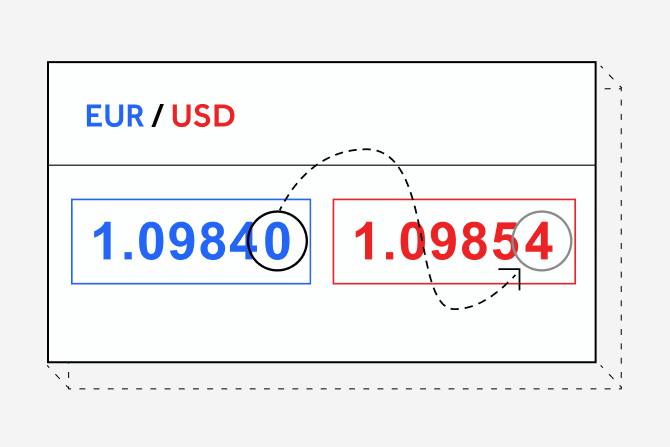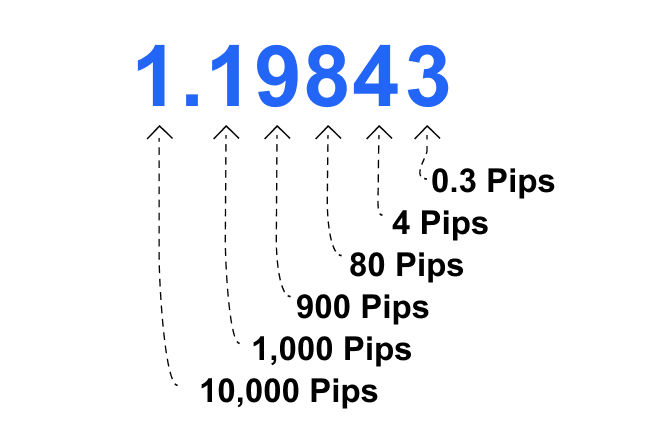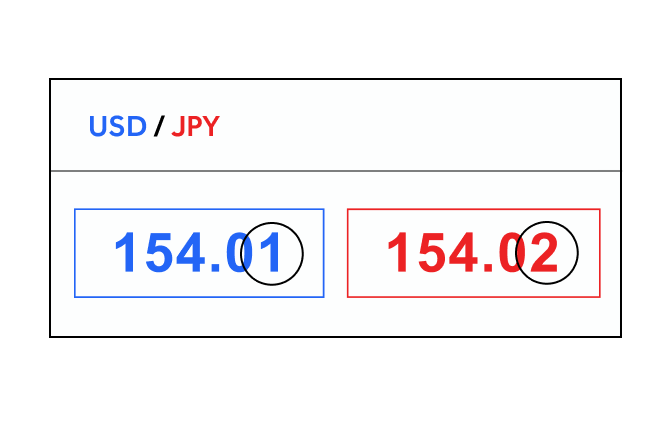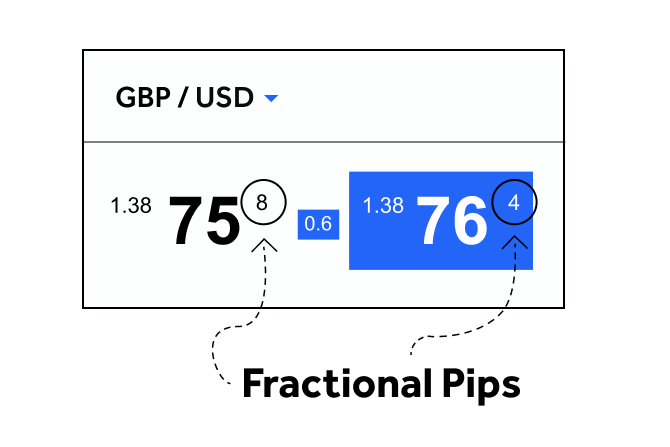CFDs are complex instruments and come with a high risk of losing money rapidly due to leverage.

76.6% of retail investor accounts lose money when trading CFDs with this provider.

You should consider whether you understand how CFDs work and whether you can afford to take the high risk of losing your money.

Choose another country or region to see content specific to your location

# What is a pip in forex trading?

#### What is a pip in forex trading? Our article explains the basics of pips and how it use it when trading FX with OANDAPip stands for “point in percentage”. A pip in forex is the smallest standardised move by which a current quote can change. As it defines the change in price between two currencies, traders calculate the spread between the bid and ask prices of the currency pair, and refer to the profit or loss that their position has made in terms of pips.

### How does a pip work?

Most currency pairs are priced out to four decimal places and the pip change is the last (fourth) decimal point. One pip, therefore, is equivalent to 1/100 of 1% or one basis point. For example, the smallest move the AUD/USD pair can make is \$0.0001, or one basis point.The Japanese yen is a notable exception, as these currency pairs are quoted to only two decimal places. To calculate a change in pips for, say USD/JPY, you have to look to the second digit after the decimal point.

With a yen currency pair such as USD/JPY, a move of 154.01 to 154.02 would be a single-pip movement. This means that if you decided to enter a long position on the pair and closed your trade as price reached 154.51, you would make a 50-pip profit (154.51 - 154.01 = 50).Let’s take a look at some examples. Let’s say you’re trading the EUR/USD currency pair. If the market were to move from 1.1600 to 1.1605, that 0.0005 increase would be a 5-pip move.

If you had entered a long position (clicking on buy), and the market moved from 1.1600 to 1.1650, and at that point you closed your trade, you would have made a profit of 50 pips (1.1600 - 1.1650 = 50). Conversely, let’s say you had placed a stop loss at 1550 and the market moved against you, dropping from 1.1600 to 1.1550. Your stop-loss order would be hit and you would have lost 50 pips (1600 - 1550 = 50).

### How to calculate profit and loss through gains and losses in pips

Example 1:

When the value of USD/CAD rises to 1.0618/1.0619, you close the trade by selling at 1.0618. Given that one pip is a movement of 0.0001, you have made a profit of 20 pips (1.0618 – 1.0598 = 0.0020 which is the equivalent of 20 pips).

The pip value in USD is (0.0001 x 100,000) ÷ 1.0568 = \$9.46.

To calculate the profit or loss on the trade, multiply the number of pips gained by the value of each pip. In this example, your profit for this trade = \$189.25 (20 x \$9.46).

Example 2:

The value of USD/CAD falls to 1.0639/1.0640 and hits your stop loss, closing the trade for a 30-pip loss.
The pip value in USD is (0.0001 x 10,000) ÷ 1.0640 = \$0.93
So you would have made a loss of \$28.19 (30 x \$0.93).

### What is a pipette?

Fractional pips, known as "pipettes", were introduced at OANDA to allow for tighter spreads. A fractional pip is equivalent to 1/10 of a pip, giving you the EUR/USD currency pair with five decimal points, while yen pairs now extend to three decimal points. Pipettes are displayed in superscript format in the quote panel.You can further build your knowledge of forex by attending free live or recorded webinars by our team of market analysts. Our professional trading instructions cover a range of strategies as well as how to use fundamental and technical analysis. You can also access a variety of tools such as the Fibonacci Retracement tool and moving averages.

### Key takeaways

• Forex currency pairs are quoted as 'pips'
• Pips are short for points in percentage
• A pip is one-hundredth of one percent, or the fourth decimal place (0.0001).
• In yen pairs, the pip is the second decimal place
• Profit and loss is calculated by measuring the total accumulation of pips, minus or positive, and multiplying that number against the trade size while taking into account leverage
• The fourth decimal place in a currency pair is the pip, and the fifth decimal place is the pipette.

Difference between leveraged and other forms of financial trading.

expand_less expand_more